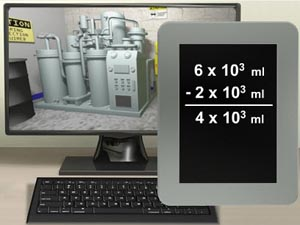StoreSeries • Module: Exponents and Scientific NotationModule: Exponents and Scientific NotationExponents and Scientific Notation

This module introduces mathematical functions using exponents and scientific notation, including a discussion of exponents, factors, radicals, and square roots. This module also explains how to identify numbers written in scientific notation. In addition, the module describes how to perform mathematical operations using exponential numbers, including addition, subtraction, multiplication, and division.

This module takes 30 minutes to complete. At the end of this module, you must obtain an 80% or higher on the Test Your Knowledge for it to be marked as complete. You have unlimited attempts and the highest attempt is recorded.

 \$10.00